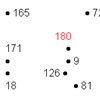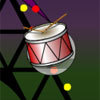Search by Topic

Resources tagged with Factors and multiples similar to Tea Cups:

Filter by: Content type:
Age range:
Challenge level:

There are 144 results

Broad Topics > Numbers and the Number System > Factors and multiplesSeven Flipped

Age 7 to 11 Challenge Level:

Investigate the smallest number of moves it takes to turn these mats upside-down if you can only turn exactly three at a time.Multiples Grid

Age 7 to 11 Challenge Level:

What do the numbers shaded in blue on this hundred square have in common? What do you notice about the pink numbers? How about the shaded numbers in the other squares?Mystery Matrix

Age 7 to 11 Challenge Level:

Can you fill in this table square? The numbers 2 -12 were used to generate it with just one number used twice.A Mixed-up Clock

Age 7 to 11 Challenge Level:

There is a clock-face where the numbers have become all mixed up. Can you find out where all the numbers have got to from these ten statements?How Old Are the Children?

Age 11 to 14 Challenge Level:

A student in a maths class was trying to get some information from her teacher. She was given some clues and then the teacher ended by saying, "Well, how old are they?"The Moons of Vuvv

Age 7 to 11 Challenge Level:

The planet of Vuvv has seven moons. Can you work out how long it is between each super-eclipse?Fractions in a Box

Age 7 to 11 Challenge Level:

The discs for this game are kept in a flat square box with a square hole for each. Use the information to find out how many discs of each colour there are in the box.Sweets in a Box

Age 7 to 11 Challenge Level:

How many different shaped boxes can you design for 36 sweets in one layer? Can you arrange the sweets so that no sweets of the same colour are next to each other in any direction?Curious Number

Age 7 to 11 Challenge Level:

Can you order the digits from 1-3 to make a number which is divisible by 3 so when the last digit is removed it becomes a 2-figure number divisible by 2, and so on?Multiply Multiples 1

Age 7 to 11 Challenge Level:

Can you complete this calculation by filling in the missing numbers? In how many different ways can you do it?Multiply Multiples 2

Age 7 to 11 Challenge Level:

Can you work out some different ways to balance this equation?Multiply Multiples 3

Age 7 to 11 Challenge Level:

Have a go at balancing this equation. Can you find different ways of doing it?Tiling

Age 7 to 11 Challenge Level:

An investigation that gives you the opportunity to make and justify predictions.A Square Deal

Age 7 to 11 Challenge Level:

Complete the magic square using the numbers 1 to 25 once each. Each row, column and diagonal adds up to 65.It Figures

Age 7 to 11 Challenge Level:

Suppose we allow ourselves to use three numbers less than 10 and multiply them together. How many different products can you find? How do you know you've got them all?Number Detective

Age 5 to 11 Challenge Level:

Follow the clues to find the mystery number.Multiplication Squares

Age 7 to 11 Challenge Level:

Can you work out the arrangement of the digits in the square so that the given products are correct? The numbers 1 - 9 may be used once and once only.Ben's Game

Age 11 to 14 Challenge Level:

Ben passed a third of his counters to Jack, Jack passed a quarter of his counters to Emma and Emma passed a fifth of her counters to Ben. After this they all had the same number of counters.Age 7 to 11 Challenge Level:

If you have only four weights, where could you place them in order to balance this equaliser?Neighbours

Age 7 to 11 Challenge Level:

In a square in which the houses are evenly spaced, numbers 3 and 10 are opposite each other. What is the smallest and what is the largest possible number of houses in the square?Round and Round the Circle

Age 7 to 11 Challenge Level:

What happens if you join every second point on this circle? How about every third point? Try with different steps and see if you can predict what will happen.Product Sudoku

Age 11 to 14 Challenge Level:

The clues for this Sudoku are the product of the numbers in adjacent squares.Cuisenaire Environment

Age 5 to 11 Challenge Level:

An environment which simulates working with Cuisenaire rods.A First Product Sudoku

Age 11 to 14 Challenge Level:

Given the products of adjacent cells, can you complete this Sudoku?Got it for Two

Age 7 to 14 Challenge Level:

Got It game for an adult and child. How can you play so that you know you will always win?A Dotty Problem

Age 7 to 11 Challenge Level:

Starting with the number 180, take away 9 again and again, joining up the dots as you go. Watch out - don't join all the dots!American Billions

Age 11 to 14 Challenge Level:

Play the divisibility game to create numbers in which the first two digits make a number divisible by 2, the first three digits make a number divisible by 3...Have You Got It?

Age 11 to 14 Challenge Level:

Can you explain the strategy for winning this game with any target?Two Primes Make One Square

Age 7 to 11 Challenge Level:

Can you make square numbers by adding two prime numbers together?Diagonal Product Sudoku

Age 11 to 16 Challenge Level:

Given the products of diagonally opposite cells - can you complete this Sudoku?Beat the Drum Beat!

Age 7 to 11 Challenge Level:

Use the interactivity to create some steady rhythms. How could you create a rhythm which sounds the same forwards as it does backwards?Odds and Threes

Age 7 to 11 Challenge Level:

A game for 2 people using a pack of cards Turn over 2 cards and try to make an odd number or a multiple of 3.Cuboids

Age 11 to 14 Challenge Level:

Find a cuboid (with edges of integer values) that has a surface area of exactly 100 square units. Is there more than one? Can you find them all?Colour Wheels

Age 7 to 11 Challenge Level:

Imagine a wheel with different markings painted on it at regular intervals. Can you predict the colour of the 18th mark? The 100th mark?Multiplication Square Jigsaw

Age 7 to 11 Challenge Level:

Can you complete this jigsaw of the multiplication square?Abundant Numbers

Age 7 to 11 Challenge Level:

48 is called an abundant number because it is less than the sum of its factors (without itself). Can you find some more abundant numbers?Crossings

Age 7 to 11 Challenge Level:

In this problem we are looking at sets of parallel sticks that cross each other. What is the least number of crossings you can make? And the greatest?Fitted

Age 7 to 11 Challenge Level:

Nine squares with side lengths 1, 4, 7, 8, 9, 10, 14, 15, and 18 cm can be fitted together to form a rectangle. What are the dimensions of the rectangle?Scoring with Dice

Age 7 to 11 Challenge Level:

I throw three dice and get 5, 3 and 2. Add the scores on the three dice. What do you get? Now multiply the scores. What do you notice?Being Collaborative - Primary Number

Age 5 to 11 Challenge Level:

Number problems at primary level to work on with others.Sets of Numbers

Age 7 to 11 Challenge Level:

How many different sets of numbers with at least four members can you find in the numbers in this box?Pebbles

Age 7 to 11 Challenge Level:

Place four pebbles on the sand in the form of a square. Keep adding as few pebbles as necessary to double the area. How many extra pebbles are added each time?Factors and Multiples Game for Two

Age 7 to 14 Challenge Level:

Factors and Multiples game for an adult and child. How can you make sure you win this game?Ip Dip

Age 5 to 11 Challenge Level:

"Ip dip sky blue! Who's 'it'? It's you!" Where would you position yourself so that you are 'it' if there are two players? Three players ...?Gran, How Old Are You?

Age 7 to 11 Challenge Level:

When Charlie asked his grandmother how old she is, he didn't get a straightforward reply! Can you work out how old she is?Which Numbers? (2)

Age 7 to 11 Challenge Level:

I am thinking of three sets of numbers less than 101. Can you find all the numbers in each set from these clues?Being Resilient - Primary Number

Age 5 to 11 Challenge Level:

Number problems at primary level that may require resilience.Surprising Split

Age 7 to 11 Challenge Level:

Does this 'trick' for calculating multiples of 11 always work? Why or why not?Which Numbers? (1)

Age 7 to 11 Challenge Level:

I am thinking of three sets of numbers less than 101. They are the red set, the green set and the blue set. Can you find all the numbers in the sets from these clues?Factor Lines

Age 7 to 14 Challenge Level:

Arrange the four number cards on the grid, according to the rules, to make a diagonal, vertical or horizontal line.